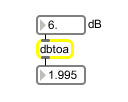# dbtoa

Convert a deciBel value to linear amplitude

## Description

dbtoa performs deciBel-gain to linear-amplitude conversion.

None.

## Messages

 bang The most currently stored linear amplitude value is sent out the outlet. int dB-gain/attenuation [int] A gain/attenuation in deciBels. The corresponding linear amplitude value is sent out the outlet. float dB-gain/attenuation [float] A gain/attenuation in deciBels. The corresponding linear amplitude value is sent out the outlet. list list of gain/attention values [list] A list of gain/attenuation values in deciBels. Corresponding linear amplitude values for each list item are sent out the outlet. set dB-gain/attenuation [float] A gain/attenuation in deciBels. The corresponding linear amplitude value is calculated but not output until a bang is received.

## Output

float: A linear amplitude value.

## Examples